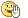#www.vustudents.ning.com

We non-commercial site working hard since 2009 to facilitate learning Read More. We can't keep up without your support. Donate.

ASSIGNMENT QUESTION:
Alec Ltd. is one of the largest electronic equipment producers in the country. It
undertakes the work according to the consumer tastes and preferences. Following data is
for the December 2012.
Cost from department - 1 Rs. 10,000
Cost added in department - 2:
Material Rs. 40,000
Labor Rs. 50,400
FOH Rs. 67,200
The quantity schedule shows that 20,000 units were received during the month from the
department - 1 and 12,000 units transferred to finished goods storeroom. 8,000 units in
process were 100% complete as to material and 60 % complete as to the conversion cost.
By using the above information, you are required to prepare/calculate:
1. Quantity schedule. 02 Marks
2. Cost accumulated in the department. 06 Marks
3. Accounting treatment / Cost apportionment. 09 Marks
4. Equivalent production units for material & conversion cost. 03 Marks
5. Per unit cost. 07 Marks
(NOTE: 03 MARKS FOR GOOD FORMATTING AND PRESENTATION)

Views: 3388

Attachments:

### Replies to This Discussion

kia smjh ni aa rhi

conversion cost kese find ki he apne?

wo conversion cost ni

wo equivalent units with refernce to coversion cost calculate kiye hn

conversion cost = direct labor + FOH (factory over head)

1. 20,000 units

2. Rs. 167,600 (Hint, it must be 100% same as given in question)

3. Cost of units manufactured = 12,000 x 9.5 = 114,000

Units in process 4,000 + 16,000 + 14,400 + 19,200 = 53,600 . So 114,000+53,600=167,600

(Hint, it must be same as given in question and calculated in req-2)

4. 20,000, 20,000, 16,800, 16800 Units

5. (10,000/20,000= 0.50), (40,000/20,000=2.00), (50,400/16,800=3.00), (67,200/16,800=4.00)

sum of all is Rs. 9.50

Best of luck. Formatting and presentation apni apni.All students must share points and also your problems.... so it can discussed and solution can prepare.

Don’t wait for solutions just participate in Discussions because after discussions a correct solution will prepare...

ye cost of units that are transfered to the finished goods he.

complete solution

Attachments:

plz koi solution upload kr doo brotherplz koi toh solution send kr doQuantity Schedule

Total unites received from department 1 are 20000 which are further processed by this department as under

United completed                               12000

Unit still in process                               8000

# Total                                                   20000

### Cost Accumulated

Cost incurred by the previous department 1                           10000

Cost incurred in department 2:

Direct Material Cost                                                               40000

Direct Labor Cost                                                                   50400

FOH                                                                                        67200

Material

Labor

FOH

# Completed units

12000

12000

12000

Ending WIP

8000(100% completed)

4800 (60% completed)

4800(60% completed)

Total

20000

16800

16800

## Cost Per Unit

Cost already incurred by previous department                       10000/20000=0.5/per unit

Direct Material Cost                                                               40000/20000=2/per unit

Direct Labor Cost                                                                   50400/16800=3/per unit

FOH                                                                                        67200/16800=4/per unit

## Cost Apportionment

Cost incurred in department 1                                                            0.5×20000=10000

Cost of completed units (12000 units completed)                  12000×9 = 108000

Cost of unit still in process:

Direct Material Cost (100% completed)                                   8000×2 = 16000

Direct Labor Cost (60% completed)                                         4800×3 = 14400

FOH (60% completed)                                                             4800×4 = 19200